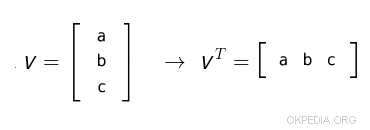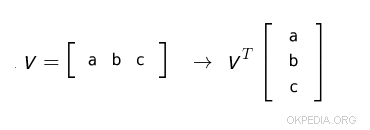# How to transpose a vector in Matlab and Octave

To transpose a vector in Matlab or Octave use transpose() function

transpose(v)

Alternatively, type the name of the vector variable by adding a quote

v'

or

v.'

The v parameter is a row or column vector (array)

In both cases the vector is transposed.

What is the transpose of a vector? After transpose, a column vector becomes a row vector.A row vector becomes a column vector.## Examples

Example 1

Create a column vector

>> V = [1;2;3]

In the column vector, the elements are on different rows

1
2
3

Type transpose(V)

>> transpose(V)

This function calculates the transpose of the vector

1 2 3

The final result is a row vector

Example 2

The same result as in the previous example can be achieved by writing V 'or V.'

>> V'

The result is a row vector with the same elements.

1 2 3

Example 3

Create a row vector

>> V = [1 2 3]

In the row vector, the elements are on the same row and in different columns

1 2 3

Now, type transpose(V)

>> transpose(V)

The result is a column vector with the same elements

1
2
3

https://how.okpedia.org/en/matlab/how-to-transpose-a-vector-in-matlab-and-octaveReport us an error or send a suggestion to improve this page